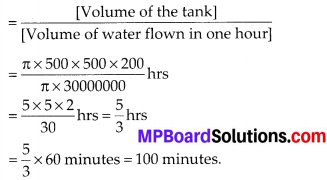In this article, we will share MP Board Class 10th Maths Book Solutions Chapter 13 Surface Areas and Volumes Ex 13.3 Pdf, These solutions are solved subject experts from the latest edition books.

MP Board Class 10th Maths Solutions Chapter 13 Surface Areas and Volumes Ex 13.3

Take π = $$\frac{22}{7}$$, unless stated otheriwise

Question 1.
A metallic sphere of radius 4.2 cm is melted and recast into the shape of a cylinder of radius 6 cm. Find the height of the cylinder.
Solution:
Radius of the sphere (r1) = 4.2 cm
∴ Volume of the sphere = $$\frac{4}{3}$$ πr13
= $$\frac{4}{3} \times \frac{22}{7} \times \frac{42}{10} \times \frac{42}{10} \times \frac{42}{10} \mathrm{cm}^{3}$$
Radius of the cylinder (r2) = 6 cm
Let h be the height of the cylinder.
∴ Volume of the cylinder = πr2h
= $$\frac{22}{7}$$ × 6 × 6 × h cm3
Since volume of the metallic sphere = Volume of the cylinder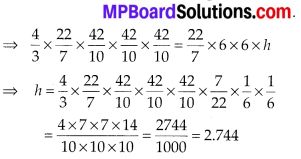Hence, the height of the cylinder is 2.744 cmQuestion 2.
Metallic spheres of radii 6 cm, 8 cm and 10 cm, respectively, are melted to form a single solid sphere. Find the radius of the resulting sphere.
Solution:
Radii of the given spheres are
r1 = 6 cm, r2 = 8 cm and r3 = 10 cm
⇒ Volume of the given spheres are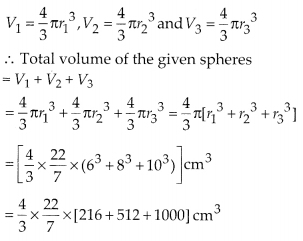= $$\frac{4}{3} \times \frac{22}{7}$$ ×  cm3
Let the radius of the new big sphere be R. Volume of the new sphere
= $$\frac{4}{3}$$ × π × R3 = $$\frac{4}{3} \times \frac{22}{7}$$ × R3
Since, the two volumes must be equal.
∴ $$\frac{4}{3} \times \frac{22}{7} \times R^{3}=\frac{4}{3} \times \frac{22}{7} \times 1728$$
⇒ R3 = 1728 ⇒ R = 12 cm
Thus, the required radius of the resulting sphere is 12 cm.

Question 3.
A 20 m deep well with diameter 7 m is dug and the earth from digging is evenly spread out to form a platform 22 m by 14 m. Find the height of the platform.
Solution:
Diameter of the cylindrical well = 7 m
⇒ Radius of the cylindrical well (r) = $$\frac{7}{2}$$ m
Depth of the well (h) = 20 m
∴ Volume = πr2h = $$\frac{22}{7} \times \frac{7}{2} \times \frac{7}{2}$$ × 20 m3
= 22 × 7 × 5 m3
⇒ Volume of the earth taken out = 22 × 7 × 5 m3
Now this earth is spread out to form a cuboidal platform having length = 22 m, breadth = 14 m
Let h be the height of the platform.
∴ Volume of the platform = 22 × 14 × h m3
Since, the two volumes must be equal
∴ 22 × 14 × h = 22 × 7 × 5
Thus, the required height of the platform is 2.5 m.Question 4.
A well of diameter 3 m is dug 14m deep. The earth taken out of it has been spread evenly all around it in shape of a circular ring of width 4 m to form an embankment. Find the height of the embankment.
Solution:
Diameter of cylindrical well = 3 m
⇒ Radius of the cylindrical well = $$\frac{3}{2}$$ m = 1.5 m
Depth of well (h) = 14 m
∴ Volume of cylindrical well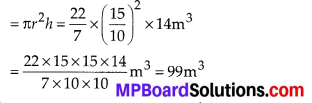Let the height of the embankment = H m.
Internal radius of the embankment (r) = 1.5 m.
External radius of the embankment (R)
= (4 + 1.5) m = 5.5 m.
∴ Volume of the embankment
= πR2H – πr2H = πH [R2 – r2]
= πH (R + r) (R – r)
= $$\frac{22}{7}$$ × H (5.5 + 1.5)(5.5 – 1.5)
= $$\frac{22}{7}$$ × H × 7 × 4m3
Since, Volume of the embankment=Volume of the cylindrical well
⇒ $$\frac{22}{7}$$ × H × 7 × 4 = 99
⇒ H = 99 × $$\frac{7}{22} \times \frac{1}{7} \times \frac{1}{4} m=\frac{9}{8} m$$ = 1.125 m
So, the required height of the embankment = 1.125 m.

Question 5.
A container shaped like a right circular cylinder having diameter 12 cm and height 15 cm is full of ice cream. The ice cream is to be filled into cones of height 12 cm and 6. diameter 6 cm, having a hemispherical shape on the top. Find the number of such cones which can be filled with ice cream.
Solution:
For the circular cylinder: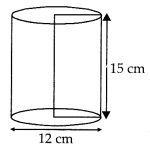Diameter = 12 cm
⇒ Radius (r) = $$\frac{12}{2}$$ = 6cm and height (h) = 15 cm
∴ Volume of circular cylinder
= πr2h = $$\frac{12}{2}$$ × 6 × 6 × 15 cm3
For conical and hemispherical part of icecream :
Diameter = 6 cm ⇒ radius (R) = 3 cm
Height of conical part (H) = 12 cm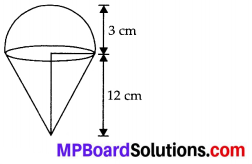Volume of ice cream cone = (Volume of the conical part) + (Volume of the hemispherical part)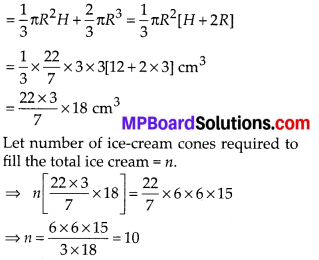Thus, the required number of cones is 10.Question 6.
How many silver coins, 1.75 cm in diameter and of thickness 2 mm, must be melted to form a cuboid of dimensions 5.5 cm × 10 cm × 3.5 cm?
Solution:
For a circular coin: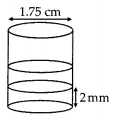Diameter = 1.75 cm
⇒ Radius (r) = $$\frac{175}{200}$$ cm
Thickness (h) = 2mm = $$\frac{2}{10}$$ cm
∴ Volume of one coin = πr2h = $$\frac{22}{7} \times\left(\frac{175}{200}\right)^{2} \times \frac{2}{10} \mathrm{cm}^{3}$$
For a cuboid: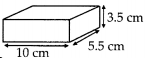Length (l) = 10 cm,
and height (h) = 3.5 cm
∴ Volume = l × b × h = 10 × $$\frac{55}{10} \times \frac{35}{10}$$ cm3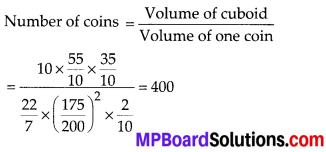Thus, the required number of coins = 400.

Question 7.
A cylindrical bucket, 32 cm high and with radius of base 18 cm, is filled with sand. This bucket is emptied on the ground and a conical heap of sand is formed. If the height of the conical heap is 24 cm, find the radius and slant height of the heap.
Solution:
For the cylindrical bucket:
Radius (r) = 18 cm and height (h) = 32 cm
Volume of cylindrical bucket = πr2h
= $$\frac{22}{7}$$ × (18)2 × 32 cm3
⇒ Volume of the sand = ($$\frac{22}{7}$$ × 18 × 18 × 32) cm3
For the conical heap:
Height (H) = 24 cm
Let radius of the base be R.
∴ Volume of conical heap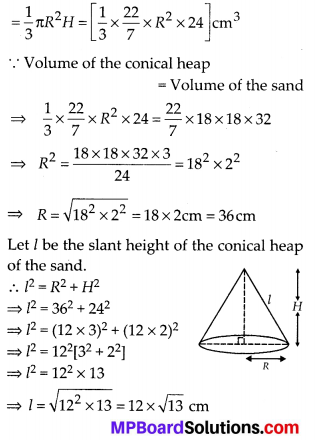Thus, the required radius = 36 cm and slant height = $$12 \sqrt{13}$$ cm.

Question 8.
Water in a canal, 6 m wide and 1.5 m deep, is flowing with a speed of 10 km/h. How much area will it irrigate in 30 minutes, if 8 cm of standing water is needed?
Solution:
Width of the canal = 6 m,
Depth of the canal = 1.5 m
Length of the water column in 1 hr = 10 km
∴ Length of the water column in 30 minutes
(i.e., $$\frac{1}{2}$$hr) = $$\frac{10}{2}$$ km = 5 km = 5000 m
∴ Volume of water flown in $$\frac{1}{2}$$ hr
= 6 × 1.5 × 5000 m3 = 6 × $$\frac{15}{10}$$ × 5000 m3
= 45000 m3
Since, the above amount (volume) of water is spread in the form of a cuboid of height
8 cm (= $$\frac{8}{100}$$ m)
Let the area of the cuboid = a
∴ Volume of the cuboid = Area × Height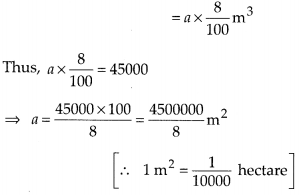= 562500 m2 = 56.25 hectares
Thus, the required area is 56.25 hectares.Question 9.
A farmer connects a pipe of internal diameter 20 cm from a canal into a cylindrical tank in her field, which is 10 m in diameter and 2 m deep. If water flows through the pipe at the rate of 3 km/h, in how much time will the tank be filled?
Solution:
Diameter of the pipe = 20 cm
⇒ Radius of the pipe (r) = $$\frac{20}{2}$$ cm = 10 cm
Since, the water flows through the pipe at 3 km/hr.
∴ Length of water column per hour(h) = 3 km
= 3 × 1000 m = 3000 × 100 cm = 300000 cm.
Length of water column per hour(h) = 3 km
Volume of water flown in one hour = πr2h
= π × 102 × 300000 cm3 = π × 30000000 cm2
Now, for the cylindrical tank :
Diameter = 10 m
⇒ Radius (R) = $$\frac{10}{2}$$ m = 5 × 100 cm = 500 cm
Height (H) = 2 m = 2 × 100 cm = 200 cm
∴ Volume of the cylindrical tank = πR2H
= π × (500)2 × 200 cm3
Now, time required to fill the tank